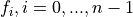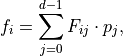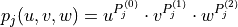# sfepy.discrete.dg.poly_spaces module¶

class sfepy.discrete.dg.poly_spaces.LegendrePolySpace(name, geometry, order, extended)[source]

Legendre hierarchical polynomials basis, over [0, 1] domain.

get_interpol_scheme()[source]

For dim > 1 returns F and P matrices according to gmsh basis specification : Let us assume that the approximation of the view’s value over an element is written as a linear combination of d basis functions(the coefficients being stored in list-of-values).

Definingwith(u, v and w being the coordinates in the element’s parameter space), then val-coef-matrix denotes the n x n matrix F and val-exp-matrix denotes the n x 3 matrix P where n is number of basis functions as calculated by get_n_el_nod.

Expects matrices to be saved in atributes coefM and expoM!

1

Remacle, J.-F., Chevaugeon, N., Marchandise, E., & Geuzaine, C. (2007). Efficient visualization of high-order finite elements. International Journal for Numerical Methods in Engineering, 69(4), 750-771. https://doi.org/10.1002/nme.1787

Returns
interp_scheme_structStruct

Struct with name of the scheme, geometry desc and P and F

get_nth_fun(n)[source]

Uses shifted Legendre polynomials formula on interval [0, 1].

Convenience function for testing

Parameters
nint
Returns
funcallable

n-th function of the legendre basis

get_nth_fun_der(n, diff=1)[source]

Returns diff derivative of nth function. Uses shifted legendre polynomials formula on interval [0, 1].

Useful for testing.

Parameters
nint
diffint

(Default value = 1)

Returns
funcallable

derivative of n-th function of the 1D legendre basis

diff derivative of the jacobi polynomials on interval [-1, 1] up to self.order + 1 at coors

Parameters
coors
alphafloat
betafloat
diffint

(Default value = 1)

Returns
valuesndarray

output shape is shape(coor) + (self.order + 1,)

Parameters
diffint

default 1

coorsarray_like

coordinates, preferably in interval [-1, 1] for which this basis is intented

Returns
valuesndarray

values at coors of all the legendre polynomials up to self.order

jacobiP(coors, alpha, beta)[source]

Values of the jacobi polynomials on interval [-1, 1] up to self.order + 1 at coors

Parameters
coorsarray_like
betafloat
alphafloat
Returns
valuesndarray

output shape is shape(coor) + (self.order + 1,)

legendreP(coors)[source]
Parameters
coorsarray_like

coordinates, preferably in interval [-1, 1] for which this basis is intented

Returns
valuesndarray

values at coors of all the legendre polynomials up to self.order

legendre_funs = [<function LegendrePolySpace.<lambda>>, <function LegendrePolySpace.<lambda>>, <function LegendrePolySpace.<lambda>>, <function LegendrePolySpace.<lambda>>, <function LegendrePolySpace.<lambda>>, <function LegendrePolySpace.<lambda>>]
class sfepy.discrete.dg.poly_spaces.LegendreSimplexPolySpace(name, geometry, order, extended=False)[source]
name = 'legendre_simplex'
class sfepy.discrete.dg.poly_spaces.LegendreTensorProductPolySpace(name, geometry, order)[source]
build_interpol_scheme()[source]

Builds F and P matrices returned by self.get_interpol_scheme.

Note that this function returns coeficients according to gmsh parametrization of Quadrangle i.e. [-1, 1] x [-1, 1] and hence the form of basis function is not the same as exhibited by the LegendreTensorProductPolySpace object which acts on parametrization [0, 1] x [0, 1].

Returns
Fndarray

coefficient matrix

Pndarray

exponent matrix

name = 'legendre_tensor_product'
sfepy.discrete.dg.poly_spaces.get_n_el_nod(order, dim, extended=False)[source]

Number of nodes per element for discontinuous legendre basis, i.e. number of iterations yielded by iter_by_order

When extended is Falsewhere n is the order and d the dimension. When extended is Truewhere n is the order and d the dimension.

Parameters
orderint

desired order of multidimensional basis

dimint

dimension of the basis

extendedbool

iterate over extended tensor product basis (Default value = False)

Returns
n_el_nodint

number of basis functions in basis

sfepy.discrete.dg.poly_spaces.iter_by_order(order, dim, extended=False)[source]

Iterates over all combinations of basis functions indexes needed to create multidimensional basis in a way that creates hierarchical basis

Parameters
orderint

desired order of multidimensional basis

dimint

dimension of the basis

extendedbool

iterate over extended tensor product basis (Default value = False)

Yields
idxtuple

containing basis function indexes, used in _combine_polyvals and _combine_polyvals_der